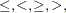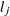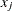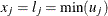### BOUNDS Statement

BOUNDS b_con [ , b_con…] ;

where b_con is given in one of the following formats:

• number operator parameter_list operator number

• number operator parameter_list

• parameter_list operator number

and operator isor.

Boundary constraints are specified with a BOUNDS statement. One- or two-sided boundary constraints are allowed. The list of boundary constraints are separated by commas. For example,

   bounds 0 <= a1-a9 X <= 1, -1 <= c2-c5;
bounds b1-b10 y >= 0;


More than one BOUNDS statement can be used. If more than one lower (upper) bound for the same parameter is specified, the maximum (minimum) of these is taken. If the maximumof all lower bounds is larger than the minimum of all upper boundsfor the same variable, the boundary constraint is replaced bydefined by the minimum of all upper bounds specified for.